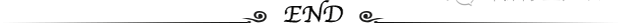# 风机静压&动压&全压，管道压力损失计算实例。

a.静压(Pi) 由于空气分子不规则运动而撞击于管壁上产生的压力称为静压计算时以绝对真空为计算零点的静压称为绝对静压。以大气压力为零点的静压称为相对静压。 空调中的空气静压均指相对静压。

b.  动压(Pb) 指空气流动时产生的压力，只要风管内空气流动就具有一定的动压。

c.全压(Pq)

d.  机外余压 机外余压的概念一般来自厂商样本

………………………………………………………………………………………

………………………………………………………………………………………

1100Pa，刚送风机的全压应不小于1390Pa，高余压一般为净化机组，风压的大 小与电机功率的选择有关一般应根据工程实际需要余压高余压并不都是好事。

150mm,4保证35m/s压。

1

R=[(λ/D)*(ν^2*γ/2)]*65

=[(0.016/0.3)*(35^2*1.2/2)]*65

=2548Pa

R1=[(λ/D)*(ν^2*γ/2)]*65

=[(0.016/0.15)*(35^2*1.2/2)]*10

=784Pa

2300mm头为90等于300mm,λ0.23

R2=λ*(ν^2*γ/2)*2

=0.23*(35^2*1.2/2)*2

=338Pa

150mm90150mm,λ0.23

R3=λ*(ν^2*γ/2)*4

=0.23*(35^2*1.2/2)*4

=676Pa

3压：

2548+784+338+676=4346Pa## 推荐产品：

18815361188